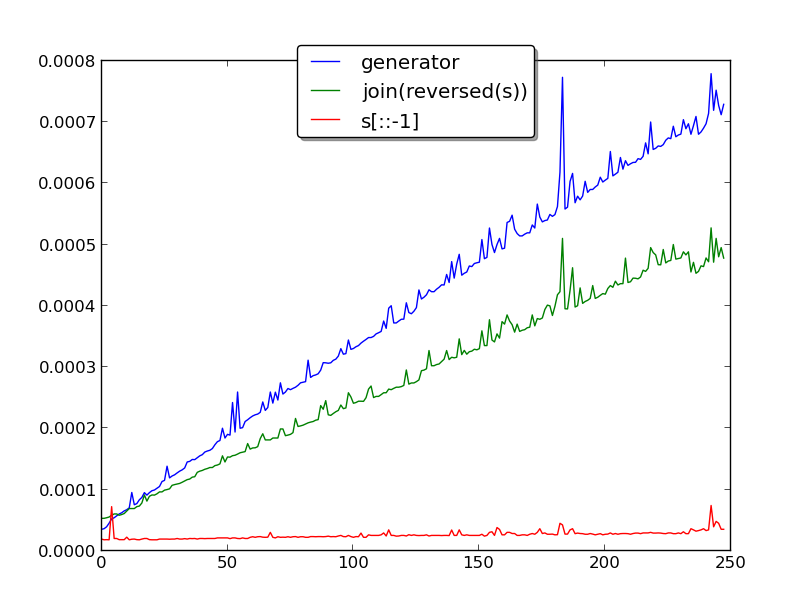# kleemans.ch

## Reverse Strings

October 3, 2012

Often there are more than one way to do things, even there may be a prefered one. Concerning string reversion, I look at three methods regarding their speed.

A simple way to reverse a given string is the following:

``````>>> s = 'Reverse'
>>> s[::-1]
'esreveR' ``````

There is also the possibility to do it with a generator:

``````>>> s = 'Teststring'
>>> ''.join([char for char in reversed(s)]) #generator
'gnirtstseT'``````

We could also use the shorter form:

``````>>> s = 'Some other String'
>>> ''.join(reversed(s))
'gnirtS rehto emoS'``````

## Speed

If we compare the speed of the three methods in seconds (y-axis), we see that with increasing string length (x-axis), the second and third methods become much slower:So it's probably better to use the shorter `s[::-1]` notation. Note that I just tested this up to strings with 250 characters, as I couldn't imagine any use case with very big strings.

## Unicode

The short method will work for Unicode too. However, remember to declare the string as unicode string:

``````>>> s = 'τεσσαρών' # wrong declaration
>>> print(s[::-1])
Ύρϱ΃σϵ΄
>>> s = u'τεσσαρών' # correct
>>> print(s[::-1])
νώρασσετ``````

An example with combined unicode characters can be found on Rosetta Code.

Code for timing (Python 2.7):

``````#!/usr/bin/python
import random
import time
import string
import matplotlib.pyplot as plt

def reverse1(s):
return s[::-1]

def reverse2(s):
return ''.join([char for char in reversed(s)])

def reverse3(s):
return ''.join(reversed(s))

def main():
t1 = list()
t2 = list()
t3 = list()
for str_len in range(5, 250):
l = list()
chars = string.letters + string.digits
#generate strings
for i in xrange(0, 100):
l.append(''.join([random.choice(chars) for i in xrange(str_len)]))

start = time.clock() #time.time()
l1 = list()
for s in l:
l1.append(reverse1(s))
t1.append(time.clock()-start)

start = time.clock()
l2 = list()
for s in l:
l2.append(reverse2(s))
t2.append(time.clock()-start)

start = time.clock()
l3 = list()
for s in l:
l3.append(reverse3(s))
t3.append(time.clock()-start)

plt.plot(t2, label="generator")
plt.plot(t3, label="join(reversed(s))")
plt.plot(t1, label="s[::-1]")
ax = plt.subplot(111)
ax.legend(loc='upper center', bbox_to_anchor=(0.5, 1.05), ncol=1, fancybox=True, shadow=True)
plt.show()

if __name__ == "__main__":
main()``````